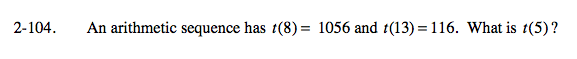### Home > A2C > Chapter 2 > Lesson 2.1.7 > Problem2-104

2-104.The general equation for an aritmetic sequence is t(n) = mn + b.

Use t(8) = 1056 to write an equation.
t(8) = m(8) + b or 1056 = 8m + b

Use t(13) = 116 to write another equation.

Solve the system of equations you wrote for m and b.

t(n) = −188n + 2560

To find t(5), substitute 5 for n in the equation that you wrote.